|

# 滴水恩 涌泉报 送礼首选“水便利”

——以“水”作春节贺礼的N种不争之理

伴随着抢票、年会、尾牙等相关图文信息开始在朋友圈中刷屏，春节正一步步走近，对于出身礼仪之邦的国人来说，长辈、家人、领导、师长、客户、下属员工、朋友……有太多的人值得我们此刻感恩、致谢，因此，拜年贺礼的选择在此刻尤为重要。

送现金？太直白，缺乏“人情味”；送大件礼品？太重，又不见得是对方生活所缺；送奢侈品、送首饰珠宝、手机数码商品？可能落入俗套……滴水之恩，当涌泉相报，在历经4000载春秋的春节“送水”或是上佳之选，既有着很好的寓意表达，又有着数种体贴入微的悉心考虑。

以水为礼的理由一：送礼就是送健康

水，无色、无味、无嗅，正是由于太过寻常，水的饮用卫生问题也是太易被忽视，长期饮用不洁净的水，会引起多种疾病，甚至威胁到生命安全。

当下，国人生活中的饮用水主要来源于自来水、饮水机、自动售水机及家用净水器，它们的饮用水品质并不容乐观。

首先，自来水是生活中最普遍应用的饮用水，绝大部分人认为把自来水煮沸便可以放心饮用。事实上，经过漫长的输水管网、水塔、水箱等设施后，进入家中的自来水中或残存着余氯和输水管道内的微生物细菌，水的品质降低，而自来水煮沸的过程实际上只能杀灭部分微生物，或无法解决残留的有机污染物和重金属离子。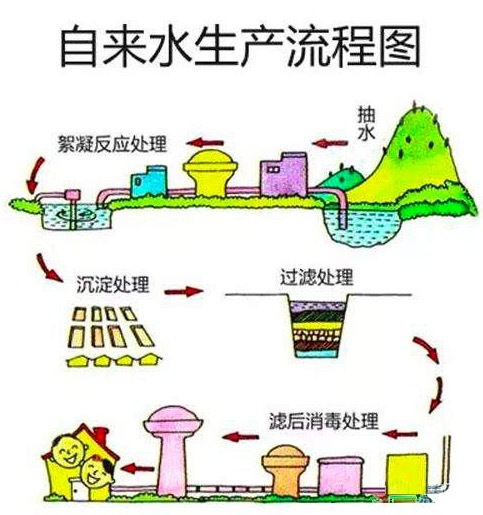其次，家中、办公室内的饮水机是一种较为常见的饮用水装备。虽然使用方便，但饮水机若不能定时进行专业清洗，会大量滋生细菌和大肠菌群、粪链球菌、肠道病毒等病菌。有报道指出，长期未清洗的饮水机，里面的细菌含量远远超过马桶里的水，而外露水龙头上滋生的病菌会比马桶座圈高2倍。再次，住家小区中的自动售水机（也有称小区净水机）虽然性价比高，但大部分机器采用的工艺是通过反渗透膜或纳滤膜生产出来的极软水。这种水在杂质被去除的同时，水中90%左右的微量元素会被过滤掉。水中的钙和镁含量也很低，长期饮用会增加心脑血管病的风险。而且，由于自动售水机在室外放置，风吹雨淋后若无定期专业清洁维护，空气中漂浮的可吸入颗粒物会附着在出水口处，并随水流进入饮用水中，这些污染肉眼不可见，更是增加了防范难度。最后，净水器虽问世多年，但由于缺少统一的标准，加之市场鱼龙混杂、售后服务不完善，因此机器质量难以保证，数年来经常被爆质量、售后、安全等负面新闻。而且，由于滤芯的更换不及时，长期积存在净水器中的杂质极容易滋生细菌和病毒，从而造成水质的污染。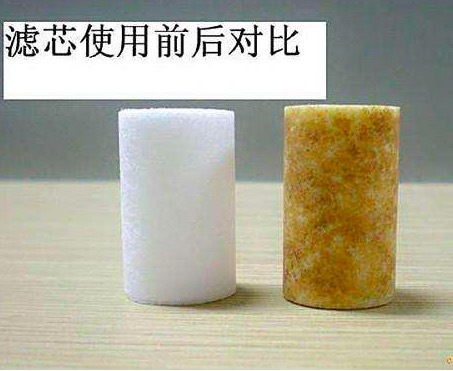此外，由于许多净水器没有安装尾水回收设施，原本可以用来洗车、浇花的水却白白流入下水道，每产出1吨纯水，要消耗数倍的原水，从而导致了资源的大量浪费。

以水为礼的理由二：送礼就要因人而异

也许，很多人认为国民开始买瓶装水、品牌水作为饮用水的行为是“消费升级”，其实，“消费升级”之于饮用水来说，绝不是消费能力、支出金额的提升，而是国民对饮用水品质需求标准的提升，是更有针对性地去选择饮用水。

近几年，为了宝宝们的健康发育，国人非常热衷于采购国外品牌奶粉，但是对于婴幼儿来说，他们的身体含水量明显高于成人，给宝宝饮用什么水、冲调奶粉用什么水的问题有所考虑吗？

有专家表示，适用于宝宝的饮食用水至少应当满足以下两点要求：1、符合商业无菌要求，不含有可能威胁婴幼儿健康的物理、化学、生物性污染；不含有致病性微生物同时不含有在常温下能在其中繁殖的非致病性微生物。2、水中含有适量的符合人体需求的矿物质，但相应矿物质的含量符合婴幼儿生理需求特点，如：钠、镁、钙、氟、锰、硫酸盐、硝酸盐等的含量相对较低，在国家限定标准之内，不会因饮水中相应矿物质的超标或全无而导致婴幼儿膳食摄入量超标或不足，不会因此给尚处于发育期的各脏器、特别是消化系统增加代谢负担甚至危害。

因此，完全去除各种矿物质的纯净水和蒸馏水，以及成人饮用的矿泉水/桶装水（往往矿物质含量较高）并不适合宝宝。通常来讲，水中的钠含量更低，易于帮助保护宝宝心脏；钙镁比例好，相互促进吸收，有效帮助宝宝生长发育；低硝酸盐、低亚硝酸盐、低氟化物能够预防婴幼儿疾病。此外，锌对生长发育旺盛的婴儿、儿童更为重要，还能够增强儿童的食欲。儿童每天约需锌0.3mg/kg体重。也就是说，只有达到商业无菌标准的低矿物元素含量的自然水才更适合宝宝。因此，爸爸妈妈一定要为宝宝选择卫生、安全且符合宝宝生理发育特点的婴儿专用饮用水，才能为宝宝健康成长打好“水基础”。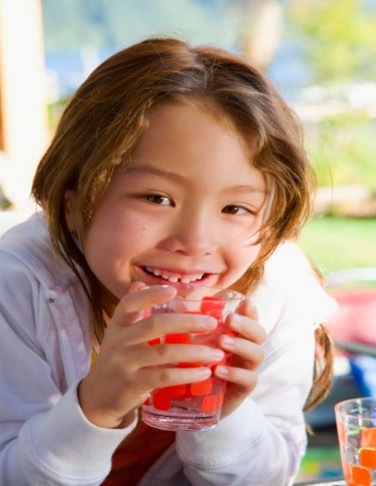而对于孕妇来说，究竟饮用什么水对胎儿、对自己有利呢？纯净水的优点在于没有细菌，没有病毒，干净卫生，但其缺点是水分子凝聚成线团状，不易被人体细胞吸收，大量饮用时，还会带走人体内有用的微量元素，从而降低人体的免疫力，容易产生疾病，对胎儿不利，所以孕妇不宜喝这类水，通常情况下，孕妇喝健康卫生的矿泉水为好，比如重碳酸钙镁型矿泉水、偏硅酸矿泉水（≥25mg/L）、锶矿泉水（≥0.2mg/L）、锌矿泉水（≥0.2mg/L）、锂矿泉水（≥0.2mg/L）等。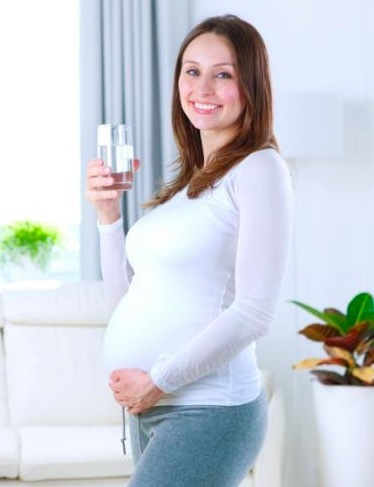此外，老年人用水也是必须要关注的问题。很多机构表示，老年人应饮用偏硅酸性水。偏硅酸是人体皮肤结缔组织、关节软骨组织和关节结缔组织中必需的元素，可增加软骨、结缔组织的弹性和强度，有助于骨的钙化，有利于骨骼钙化和防治骨质疏松。同时，偏硅酸对人体主动脉硬化具有软化作用，对心脏病、高血压、动脉硬化、神经功能紊乱、胃炎及消化道溃疡具有一定的保健作用，还可有效降低人体内的铝元素水平，能预防并改善老年痴等症状。老年人也可饮用含锶的矿泉水，锶能减少人体对钠的吸收，增加钠的排泄，对高血压、高血脂、高血糖、心血管疾病、动脉硬化等疾病有一些缓解作用。此外，也可饮用含硒的矿泉水（≥0.01mg/L），硒元素具有抗氧化,抗癌抑瘤等作用，是人体必不可缺的珍惜微量元素，经常饮用硒矿泉水，能够增加细胞活性，有效的预防肿瘤和癌症。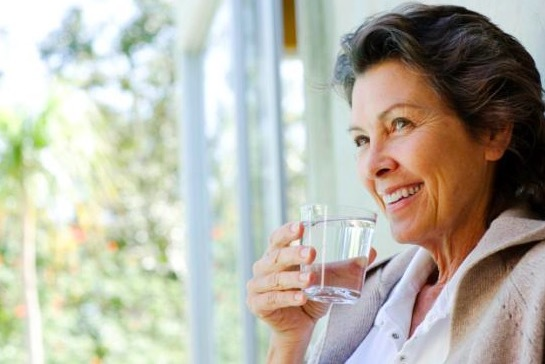除去这些特定人群外，一天大约花费8个小时以上的时间进行工作的白领，喝水也是需要有所考虑的。比如，对于那些在疲劳和压力下工作的人们来说，他们消耗的能量和电解质比较多,天然矿泉水胜似良药。天然矿泉水除了无污染的特质之外，水中含有丰富的常量和微量元素，钾、钠、钙、镁等矿物盐则有助于补充能量，增添活力。对于女性白领，经常饮用富含偏硅酸的矿泉水，可使皮肤保持光泽、白皙、细嫩、有弹性，把偏硅酸矿泉水直接喷在脸上的美容法也越来越被诸多爱美女性所青睐。

而对于一个企业来说，高层领导、企业领袖的饮用水选择要是值得注意的问题。也许，在不铺张浪费的大环境下，对比各跨国巨头企业强制要求员工差旅时要住5星级宾馆的规定，让高阶管理者不再局限于桶装水，而是可以按自己喜好，或是按照营养元素去选择一些国内外高规格品牌瓶装水更趋合理。同时，高阶领导者在会客时也能适当地凸显身份，提升会客档次。以水为礼的理由三：送礼就是送时尚

“水中毒症”一词是我们上学时代学到的知识。其实，除了上述不同人群的饮用水需求界定划分外，人们对于饮用水的场景也是有着一定的学问的。

比如泡茶时，茶叶中的茶多酚具有很强的抗氧化能力，但它也有脆弱的一面，在碱性环境下容易发生氧化聚合，颜色加深，并且丧失一定程度的抗氧化能力。所以泡茶应当使用弱酸性水，且其中几乎不含杂质，茶汤晶莹剔透，香味也更加醇正，没有其他的杂味，口感鲜醇。而不同类别的茶对于适合的冲调水的选择也不同。比如，绿茶用软水（TDS，即Total dissolved solids，总溶解固体，TDS值越低，水越软，反之越硬）来泡，红茶要用硬水来泡，乌龙半软不硬的水最好……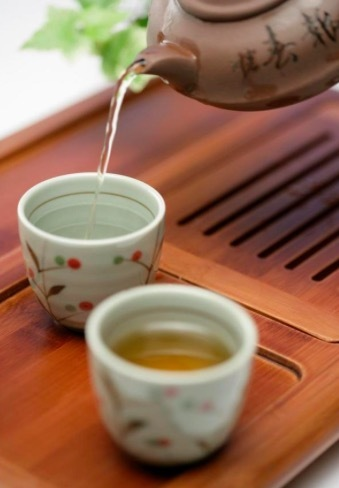以水为礼的理由四：送礼就是送便捷

事实上，当下人们对饮用水的需求已从基础属性和认知属性上，向着更细分、更有针对性的方向发展了，健康、科学地饮水将推动着国人进入饮水3.0时代。也正是如此，作为国内需求型智慧电商的领导者——消费宝商城（xiaofeibao.com）在新春来临之季，为满足中高端用户饮用水需求，通过与百余家中外知名饮用水品牌企业的深度合作，推出了以双向O2O的模式为用户提供“专业、安全、实惠、便利”的“贴身水管家”式专业饮用水服务平台。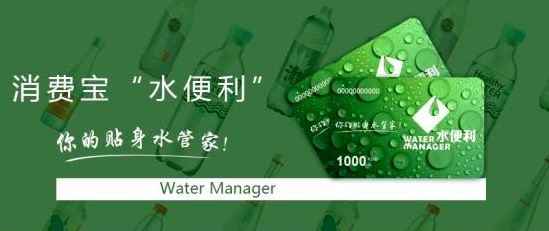消费宝水便利

水便利卡是消费宝商城所辖的水便利平台的专项服务卡，从产地、水源、功能、适合人群、PH值等诸多维度科学并严格遴选全品类、全规格的中外百余品牌的饮用水商品，种类、规格齐全，能够满足人们对水的差异化需求。同时，水便利通过减少中间商环节，保证商品物美价廉的同时，经权威机构进行评测和推荐，帮助用户喝对水、喝好水。而且，水便利卡还整合了全国数十万个网点，实现线上、线下销售、物流配送、服务一体化，为用户提供更加便捷的服务。

以“水”为礼，脱俗又因循古训，既是日常生活所需的廉洁之礼，关爱有加，合情合理，又避免了送“体检卡”引起的歧义。有网友调侃道，“五行中，水通常象征着财富，今年又恰逢农历鸡年，鸡属金，金生水，所以鸡年近水寓意吉祥”。因此，于情、于理、于吉祥，

在新年之际“以水为礼”则显得更加别出心裁，不落俗套。

`声明：本文由入驻焦点开放平台的作者撰写，除焦点官方账号外，观点仅代表作者本人，不代表焦点立场错误信息举报电话： 400-099-0099，邮箱：jubao@vip.sohu.com，或点此进行意见反馈，或点此进行举报投诉。`A B C D E F G H J K L M N P Q R S T W X Y Z
A - B - C - D - E
• A
• 鞍山
• 安庆
• 安阳
• 安顺
• 安康
• 澳门
• B
• 北京
• 保定
• 包头
• 巴彦淖尔
• 本溪
• 蚌埠
• 亳州
• 滨州
• 北海
• 百色
• 巴中
• 毕节
• 保山
• 宝鸡
• 白银
• 巴州
• C
• 承德
• 沧州
• 长治
• 赤峰
• 朝阳
• 长春
• 常州
• 滁州
• 池州
• 长沙
• 常德
• 郴州
• 潮州
• 崇左
• 重庆
• 成都
• 楚雄
• 昌都
• 慈溪
• 常熟
• D
• 大同
• 大连
• 丹东
• 大庆
• 东营
• 德州
• 东莞
• 德阳
• 达州
• 大理
• 德宏
• 定西
• 儋州
• 东平
• E
• 鄂尔多斯
• 鄂州
• 恩施
F - G - H - I - J
• F
• 抚顺
• 阜新
• 阜阳
• 福州
• 抚州
• 佛山
• 防城港
• G
• 赣州
• 广州
• 桂林
• 贵港
• 广元
• 广安
• 贵阳
• 固原
• H
• 邯郸
• 衡水
• 呼和浩特
• 呼伦贝尔
• 葫芦岛
• 哈尔滨
• 黑河
• 淮安
• 杭州
• 湖州
• 合肥
• 淮南
• 淮北
• 黄山
• 菏泽
• 鹤壁
• 黄石
• 黄冈
• 衡阳
• 怀化
• 惠州
• 河源
• 贺州
• 河池
• 海口
• 红河
• 汉中
• 海东
• 怀来
• I
• J
• 晋中
• 锦州
• 吉林
• 鸡西
• 佳木斯
• 嘉兴
• 金华
• 景德镇
• 九江
• 吉安
• 济南
• 济宁
• 焦作
• 荆门
• 荆州
• 江门
• 揭阳
• 金昌
• 酒泉
• 嘉峪关
K - L - M - N - P
• K
• 开封
• 昆明
• 昆山
• L
• 廊坊
• 临汾
• 辽阳
• 连云港
• 丽水
• 六安
• 龙岩
• 莱芜
• 临沂
• 聊城
• 洛阳
• 漯河
• 娄底
• 柳州
• 来宾
• 泸州
• 乐山
• 六盘水
• 丽江
• 临沧
• 拉萨
• 林芝
• 兰州
• 陇南
• M
• 牡丹江
• 马鞍山
• 茂名
• 梅州
• 绵阳
• 眉山
• N
• 南京
• 南通
• 宁波
• 南平
• 宁德
• 南昌
• 南阳
• 南宁
• 内江
• 南充
• P
• 盘锦
• 莆田
• 平顶山
• 濮阳
• 攀枝花
• 普洱
• 平凉
Q - R - S - T - W
• Q
• 秦皇岛
• 齐齐哈尔
• 衢州
• 泉州
• 青岛
• 清远
• 钦州
• 黔南
• 曲靖
• 庆阳
• R
• 日照
• 日喀则
• S
• 石家庄
• 沈阳
• 双鸭山
• 绥化
• 上海
• 苏州
• 宿迁
• 绍兴
• 宿州
• 三明
• 上饶
• 三门峡
• 商丘
• 十堰
• 随州
• 邵阳
• 韶关
• 深圳
• 汕头
• 汕尾
• 三亚
• 三沙
• 遂宁
• 山南
• 商洛
• 石嘴山
• T
• 天津
• 唐山
• 太原
• 通辽
• 铁岭
• 泰州
• 台州
• 铜陵
• 泰安
• 铜仁
• 铜川
• 天水
• 天门
• W
• 乌海
• 乌兰察布
• 无锡
• 温州
• 芜湖
• 潍坊
• 威海
• 武汉
• 梧州
• 渭南
• 武威
• 吴忠
• 乌鲁木齐
X - Y - Z
• X
• 邢台
• 徐州
• 宣城
• 厦门
• 新乡
• 许昌
• 信阳
• 襄阳
• 孝感
• 咸宁
• 湘潭
• 湘西
• 西双版纳
• 西安
• 咸阳
• 西宁
• 仙桃
• 西昌
• Y
• 运城
• 营口
• 盐城
• 扬州
• 鹰潭
• 宜春
• 烟台
• 宜昌
• 岳阳
• 益阳
• 永州
• 阳江
• 云浮
• 玉林
• 宜宾
• 雅安
• 玉溪
• 延安
• 榆林
• 银川
• Z
• 张家口
• 镇江
• 舟山
• 漳州
• 淄博
• 枣庄
• 郑州
• 周口
• 驻马店
• 株洲
• 张家界
• 珠海
• 湛江
• 肇庆
• 中山
• 自贡
• 资阳
• 遵义
• 昭通
• 张掖
• 中卫

1室1厅1厨1卫1阳台

1
2
3
4
5

0
1
2

1

1

0
1
2
3报名成功，资料已提交审核A B C D E F G H J K L M N P Q R S T W X Y Z
A - B - C - D - E
• A
• 鞍山
• 安庆
• 安阳
• 安顺
• 安康
• 澳门
• B
• 北京
• 保定
• 包头
• 巴彦淖尔
• 本溪
• 蚌埠
• 亳州
• 滨州
• 北海
• 百色
• 巴中
• 毕节
• 保山
• 宝鸡
• 白银
• 巴州
• C
• 承德
• 沧州
• 长治
• 赤峰
• 朝阳
• 长春
• 常州
• 滁州
• 池州
• 长沙
• 常德
• 郴州
• 潮州
• 崇左
• 重庆
• 成都
• 楚雄
• 昌都
• 慈溪
• 常熟
• D
• 大同
• 大连
• 丹东
• 大庆
• 东营
• 德州
• 东莞
• 德阳
• 达州
• 大理
• 德宏
• 定西
• 儋州
• 东平
• E
• 鄂尔多斯
• 鄂州
• 恩施
F - G - H - I - J
• F
• 抚顺
• 阜新
• 阜阳
• 福州
• 抚州
• 佛山
• 防城港
• G
• 赣州
• 广州
• 桂林
• 贵港
• 广元
• 广安
• 贵阳
• 固原
• H
• 邯郸
• 衡水
• 呼和浩特
• 呼伦贝尔
• 葫芦岛
• 哈尔滨
• 黑河
• 淮安
• 杭州
• 湖州
• 合肥
• 淮南
• 淮北
• 黄山
• 菏泽
• 鹤壁
• 黄石
• 黄冈
• 衡阳
• 怀化
• 惠州
• 河源
• 贺州
• 河池
• 海口
• 红河
• 汉中
• 海东
• 怀来
• I
• J
• 晋中
• 锦州
• 吉林
• 鸡西
• 佳木斯
• 嘉兴
• 金华
• 景德镇
• 九江
• 吉安
• 济南
• 济宁
• 焦作
• 荆门
• 荆州
• 江门
• 揭阳
• 金昌
• 酒泉
• 嘉峪关
K - L - M - N - P
• K
• 开封
• 昆明
• 昆山
• L
• 廊坊
• 临汾
• 辽阳
• 连云港
• 丽水
• 六安
• 龙岩
• 莱芜
• 临沂
• 聊城
• 洛阳
• 漯河
• 娄底
• 柳州
• 来宾
• 泸州
• 乐山
• 六盘水
• 丽江
• 临沧
• 拉萨
• 林芝
• 兰州
• 陇南
• M
• 牡丹江
• 马鞍山
• 茂名
• 梅州
• 绵阳
• 眉山
• N
• 南京
• 南通
• 宁波
• 南平
• 宁德
• 南昌
• 南阳
• 南宁
• 内江
• 南充
• P
• 盘锦
• 莆田
• 平顶山
• 濮阳
• 攀枝花
• 普洱
• 平凉
Q - R - S - T - W
• Q
• 秦皇岛
• 齐齐哈尔
• 衢州
• 泉州
• 青岛
• 清远
• 钦州
• 黔南
• 曲靖
• 庆阳
• R
• 日照
• 日喀则
• S
• 石家庄
• 沈阳
• 双鸭山
• 绥化
• 上海
• 苏州
• 宿迁
• 绍兴
• 宿州
• 三明
• 上饶
• 三门峡
• 商丘
• 十堰
• 随州
• 邵阳
• 韶关
• 深圳
• 汕头
• 汕尾
• 三亚
• 三沙
• 遂宁
• 山南
• 商洛
• 石嘴山
• T
• 天津
• 唐山
• 太原
• 通辽
• 铁岭
• 泰州
• 台州
• 铜陵
• 泰安
• 铜仁
• 铜川
• 天水
• 天门
• W
• 乌海
• 乌兰察布
• 无锡
• 温州
• 芜湖
• 潍坊
• 威海
• 武汉
• 梧州
• 渭南
• 武威
• 吴忠
• 乌鲁木齐
X - Y - Z
• X
• 邢台
• 徐州
• 宣城
• 厦门
• 新乡
• 许昌
• 信阳
• 襄阳
• 孝感
• 咸宁
• 湘潭
• 湘西
• 西双版纳
• 西安
• 咸阳
• 西宁
• 仙桃
• 西昌
• Y
• 运城
• 营口
• 盐城
• 扬州
• 鹰潭
• 宜春
• 烟台
• 宜昌
• 岳阳
• 益阳
• 永州
• 阳江
• 云浮
• 玉林
• 宜宾
• 雅安
• 玉溪
• 延安
• 榆林
• 银川
• Z
• 张家口
• 镇江
• 舟山
• 漳州
• 淄博
• 枣庄
• 郑州
• 周口
• 驻马店
• 株洲
• 张家界
• 珠海
• 湛江
• 肇庆
• 中山
• 自贡
• 资阳
• 遵义
• 昭通
• 张掖
• 中卫• 手机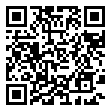• 分享
• 设计
免费设计
• 计算器
装修计算器
• 入驻
合作入驻
• 联系
联系我们
• 置顶
返回顶部